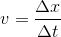# High School Physics : Understanding Independent and Dependent Variables

## Example Questions

### Example Question #1 : Understanding Independent And Dependent Variables

A scientist measures how far a particle travels within a given amount of time. Every second she measures how far it has gone, and creates a graph of her results. What is the independent variable in this experiment?

Neither variable is independent

It will depend on the shape of the graph

Time

Distance

Both variables are independent

Time

Explanation:

An independent variable is manipulated by the experimenter. Any changes made are predictable. The dependent variable reacts to changes made to the independent variable. Its changes are not controlled by the experimenter and can be hard to predict.

In this particular experiment, the scientist is measuring how the particle's distance changes over a given time. She is able to control the amount of time that she measures, but is only able to observe the distance traveled.

In the graph, the independent variable will be graphed on the x-axis.

### Example Question #2 : Understanding Independent And Dependent Variables

When looking at velocity in terms of distance and time, what is the independent variable?

Acceleration

Distance

Time

Velocity

Displacement

Time

Explanation:

There are a few ways to think of this question. The first is to imagine you are graphing velocity. Since the equation is, the displacement would be on the y-axis and time would be on the x-axis. The x-axis is going to be where we put our independent variable.

The other way to think of this is to ask yourself what our "inputs" and "outputs" would be if we were measuring velocity. Imagine you're walking down the street and you record how far you travel every second. The time is what you are "inputting" and your distance travelled is your "output."

### Example Question #1 : Understanding Independent And Dependent Variables

You are looking at a graph of the motion of a vehicle. On the y-axis is displacement and on the x-axis is time. Which of the following is the independent variable?

Displacement

Velocity

Acceleration

Time

Distance

Time

Explanation:

Independent variables are predetermined by the experimenter and can be manipulated to change the measured dependent variable. Independent variables are generally graphed on the x-axis, while dependent variables are generally graphed on the y-axis.

In this question, time is the independent variable and displacement is the dependent variable. The experimenter can select sampling times, but cannot necessarily predict the displacement that will be measured at each point. This defines time as the independent variable.

### Example Question #1 : Understanding Independent And Dependent Variables

Where is the independent variable most commonly displayed on a graph?

Sometimes along the x-axis and sometimes along the y-axis

Along the y-axis

The independent variable is not displayed

Along both the x- and y-axes

Along the x-axis